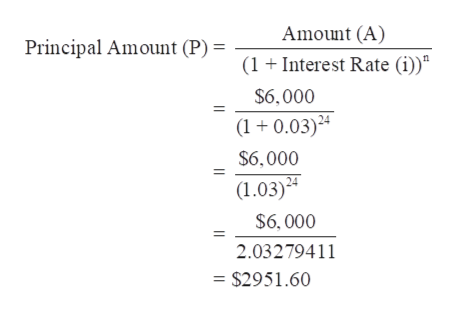# Use the compound interest formula A = P(1 + i)n to find the indicated values A = \$6,000; i = 0.03; n = 24; P =?

Question
1 views

Use the compound interest formula A = P(1 + i)n to find the indicated values A = \$6,000; = 0.03; n = 24; P =?

check_circle

Step 1

Compounded Interest:

It is the method for computing interest at the initial amount of principal which includes the previous period’s accumulated interest. It can be compounded annually, half-yearly, quarterly and daily. The rate of compounding interest depends on the frequency of compounding.

Step 2

Given,

Amount (A) = \$6,000

Interest Rate (i) = 0.03

Number of times compounded (n...help_outlineImage TranscriptioncloseAmount (A) Principal Amount (P) (1Interest Rate (i)) \$6,000 (1 0.03) \$6,000 (1.03) \$6, 000 2.03279411 = \$2951.60 fullscreen

### Want to see the full answer?

See Solution

#### Want to see this answer and more?

Solutions are written by subject experts who are available 24/7. Questions are typically answered within 1 hour.*

See Solution
*Response times may vary by subject and question.
Tagged in